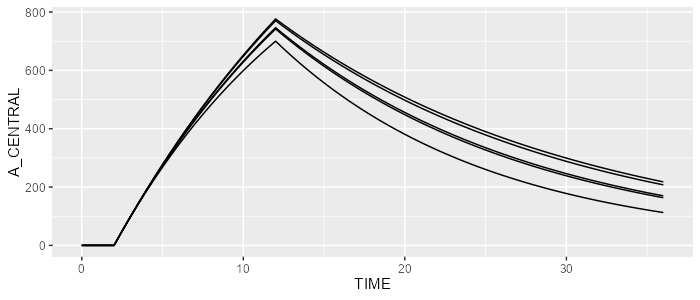# Play with compartment properties

### Prerequisite

For this vignette, please load the campsismod package and load the minimalist model that we have created in the first vignette.

library(campsismod)
model <- read.campsis("resources/minimalist_model/")

### Create new compartment properties

Let’s invent a very basic scenario: we would like to infuse 1000 into the central compartment with a fixed rate of 100 and a fixed lag time of 2.
First, we’re going to delete the initial condition that we had in the minimalist model. This is done as follows:

model_ <- model %>% delete(InitialCondition(compartment=1))
model_
## [MAIN]
## K=THETA_K*exp(ETA_K) # Elimination constant
##
## [ODE]
## d/dt(A_CENTRAL)=-K*A_CENTRAL
##
##
## THETA's:
##   name index value   fix
## 1    K     1  0.06 FALSE
## OMEGA's:
##   name index index2 value   fix type same
## 1    K     1      1    15 FALSE  cv%   NA
## SIGMA's:
## # A tibble: 0 × 0
## No variance-covariance matrix
##
## Compartments:
## A_CENTRAL (CMT=1)

This is strictly equal as doing (if you prefer working with compartment names):

model <- model %>% delete(InitialCondition(compartment= model %>% getCompartmentIndex("CENTRAL")))

We can now add a fixed rate for all infusions that go into the central compartment:

model <- model %>% add(InfusionRate(compartment=1, "100"))

Finally, let’s now add a constant lag time:

model <- model %>% add(LagTime(compartment=1, "2"))

OK, this is how our model looks like now:

model
## [MAIN]
## K=THETA_K*exp(ETA_K) # Elimination constant
##
## [ODE]
## d/dt(A_CENTRAL)=-K*A_CENTRAL
##
## [LAG]
## A_CENTRAL=2
##
## [RATE]
## A_CENTRAL=100
##
##
## THETA's:
##   name index value   fix
## 1    K     1  0.06 FALSE
## OMEGA's:
##   name index index2 value   fix type same
## 1    K     1      1    15 FALSE  cv%   NA
## SIGMA's:
## # A tibble: 0 × 0
## No variance-covariance matrix
##
## Compartments:
## A_CENTRAL (CMT=1)

### Simulate our model

Let’s now simulate a few individuals and show A_CENTRAL, i.e., the amount of drug in the central compartment.

First, we need to define an infusion of 1000 in a CAMPSIS dataset, as well as the observations times.

library(campsis)
dataset <- Dataset(5) %>%
add(Observations(seq(0,36,by=0.5)))

Then, we can run the simulation.

results <- model %>% simulate(dataset=dataset, seed=1)
spaghettiPlot(results, "A_CENTRAL")### A couple of useful functions in action

As previously, let’s demonstrate the use of a couple of interesting functions:

Check the existence of a compartment:

model %>% contains(Compartment(1)) 
##  TRUE
# Or equivalenty:
model %>% contains(Compartment(model %>% getCompartmentIndex("CENTRAL")))
##  TRUE

Check the existence of a property:

model %>% contains(InfusionRate(1))
##  TRUE
model %>% contains(InfusionDuration(1)) 
##  FALSE

Find a compartment:

model %>% find(Compartment(1)) 
## A_CENTRAL (CMT=1)

Find a compartment property:

model %>% find(InfusionRate(1)) 
## RATE (CMT=1): 100

Replace a compartment property:

model %>% replace(InfusionRate(1, "200")) # Previous value of 100 is overridden
## [MAIN]
## K=THETA_K*exp(ETA_K) # Elimination constant
##
## [ODE]
## d/dt(A_CENTRAL)=-K*A_CENTRAL
##
## [LAG]
## A_CENTRAL=2
##
## [RATE]
## A_CENTRAL=200
##
##
## THETA's:
##   name index value   fix
## 1    K     1  0.06 FALSE
## OMEGA's:
##   name index index2 value   fix type same
## 1    K     1      1    15 FALSE  cv%   NA
## SIGMA's:
## # A tibble: 0 × 0
## No variance-covariance matrix
##
## Compartments:
## A_CENTRAL (CMT=1)

Interestingly, the name of a compartment can be replaced as follows:

model %>% replace(Compartment(1, name="CENT")) %>%
delete(Ode("A_CENTRAL")) %>%
add(Ode("A_CENT", "-K*A_CENT"))
## [MAIN]
## K=THETA_K*exp(ETA_K) # Elimination constant
##
## [ODE]
## d/dt(A_CENT)=-K*A_CENT
##
## [LAG]
## A_CENT=2
##
## [RATE]
## A_CENT=100
##
##
## THETA's:
##   name index value   fix
## 1    K     1  0.06 FALSE
## OMEGA's:
##   name index index2 value   fix type same
## 1    K     1      1    15 FALSE  cv%   NA
## SIGMA's:
## # A tibble: 0 × 0
## No variance-covariance matrix
##
## Compartments:
## A_CENT (CMT=1)